# SAS/STAT Examples

## Bayesian Multinomial Model for Ordinal Data

Contents | SAS Program | PDF

## Overview

This example illustrates how to fit a Bayesian multinomial model by using the built-in mutinomial density function (MULTINOM) in the MCMC procedure for categorical response data that are measured on an ordinal scale. By using built-in multivariate distributions, PROC MCMC can efficiently sample constrained multivariate parameters with random walk Metropolis algorithm. The example also demonstrates how use the MCMC procedure to compute posterior means, credible intervals, and posterior distributions of the parameters and odds ratios for the multinomial model.

## Analysis

Researchers study the results of a taste test on three different brands of ice cream. They want to assess the testers’ preference of the three brands. The taste of each brand is rated on a five-point scale from very good to very bad. the five points correspond to response variables Y1 through Y5, where Y1 represents very good and Y5 represents very bad. Response variables contain the number of taste testers who rate each brand in each category. The very bad taste level (Y5) is used as the reference response level. Two dummy variables, BRAND1 and BRAND2, are created to indicate Brands 1 and 2, respectively. Brand 3 is set as the reference level in this example and is represented in the data set when both of the dummy variables equal zero.

The following statements create the ICECREAM data set:

```data icecream;
input y1-y5 brand;
if brand = 1 then brand1 = 1;
else brand1 = 0;
if brand = 2 then brand2 = 1;
else brand2 = 0;
keep y1-y5 brand1 brand2;
datalines;
70 71 151 30 46 1
20 36 130 74 70 2
50 55 140 52 50 3
;
```

## Bayesian Multinomial Model

Multinomial ordinal models occur frequently in applications such as food testing, survey response, or anywhere order matters in the categorical response. Categorical data with an ordinal response correspond to multinomial models based on cumulative response probabilities (McCullagh and Nelder; 1989). In this data set, the ordered response variable is the taste tester’s rating for a brand of ice cream.

Let the random variable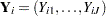for brand, and let response level, be from an multinomial ordinal model with mutually exclusive, discrete response levels and probability mass function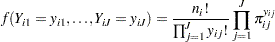where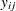represents the number of people fromth brand in theth response level. For the grouped data, letdenote the number of testers who taste theth brand of ice cream and let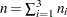. Letdenote the probability that the response of brandfalls into theth response level, and let. Let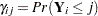denote the corresponding cumulative probability that the response falls in theth level or below, so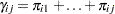. The transformed cumulative probabilities are linear functions of the covariates written as, whererefers to the logit link function,represents the effects for the covariates, and. Let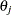represent the baseline value of the transformed cumulative probability for categorysuch that the constraint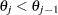holds for all(Albert and Chib; 1993). Then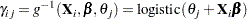(1)

and the group probabilities for theth levels are as follows: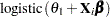(2)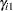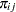(3)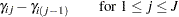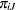(4)

The likelihood function for the counts and corresponding covariates is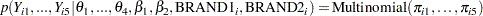(5)

where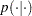denotes a conditional probability density. The multinomial density is evaluated at the specified value of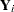and the corresponding probabilities, which are defined in Equation 2 through 4.

There are six parameters in the likelihood: the intercepts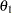throughand the regression parametersand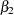that correspond to the relative Brand 1 and 2 effects, respectively.

Suppose the following prior distributions are placed on the six parameters, whereindicates a prior distribution andindicates a conditional prior distribution: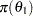(6)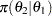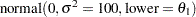(7)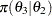(8)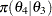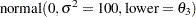(9)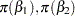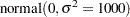(10)

The joint prior distribution ofthroughis the product of Equation 6 through 9. The prior distributions in Equation 7 through 9 represent truncated normal distributions with mean 0, variance 100, and the designated lower bound. The lower bound ensures that the order restriction onis sustained.

Using Bayes’ theorem, the likelihood function and prior distributions determine the posterior distribution of the parameters as follows: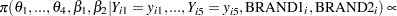PROC MCMC obtains samples from the desired posterior distribution. You do not need to specify the exact form of the posterior distribution.

The odds ratio for comparing one brand to another can be written as(11)

for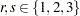. The odds ratio is useful for interpreting how the taste preference for the different brands of ice cream cpmpares. For this example, Brand 3 is set as the reference level, which implies that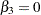.

The following SAS statements fit the Bayesian multinomial ordinal model. The PROC MCMC statement invokes the procedure and specifies the input data set. The NBI= option specifies the number of burn-in iterations. The NMC= option specifies the number of posterior simulation iterations. The THIN=10 option specifies that one of every 10 samples is kept. The SEED= option specifies a seed for the random number generator (the seed guarantees the reproducibility of the random stream). The PROPCOV=QUANEW option uses the estimated inverse Hessian matrix as the initial proposal covariance matrix. The MONITOR= option outputs analysis on selected symbols of interest in the program.

```ods graphics on;
proc mcmc data=icecream nbi=10000 nmc=25000 thin=10 seed=1181
propcov=quanew monitor=(beta1 beta2 or12 or13 or23);
array data y1 y2 y3 y4 y5;
array theta;
array gamma;
array pi;

parms theta1-theta4 beta1 beta2;
prior theta1 ~ normal(0,var=100);
prior theta2 ~ normal(0,var=100,lower=theta1);
prior theta3 ~ normal(0,var=100,lower=theta2);
prior theta4 ~ normal(0,var=100,lower=theta3);
prior beta: ~ normal(0,var=1000);

mu = beta1*brand1 + beta2*brand2;
do j = 1 to 4;
gamma[j] = logistic(theta[j] + mu);
if j>=2  then pi[j]=gamma[j]-gamma[j-1];
end;
pi1 = gamma1;
pi5 = 1 - sum(of pi1-pi4);

model data~multinom(pi);

beginnodata;
or12 = exp(beta1-beta2);
or13 = exp(beta1);
or23 = exp(beta2);
endnodata;
run;
ods graphics off;
```

Each of the ARRAY statements associate a name with a list of variables and constants. The first ARRAY statement declare the data array for response variables Y1 through Y5. The second ARRAY statement specifies names for the intercept parameters. The third ARRAY statement contains the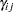parameters and the last ARRAY statement contains theparameters.

The PARMS statement puts allandparameters in a single block. The PRIOR statements specify priors for the parameters as given in Equations 6 through 10.

The MU assignment statement calculates. The DO loop and coinciding GAMMA assignment statements calculatefor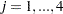as in Equation 1. The five PI assignment statements calculate the individual probabilities that an observation falls into theth response level as in Equation 2 to Equation 4.

For SAS/STAT 9.3 and later, the MODEL statement supports the multinomial density function (MULTINOM). Hence, it is used to construct the likelihood function for the response variables Y1 through Y5 and the model parametersas in Equation 5. Note that MULTINOM is not supported by the PRIOR and HYPERPRIOR statements. However, you can still declare multinomial prior by using the GENERAL function and SAS programming statements that use LOGMPDFMULTINOM.

The statements within the BEGINNODATA and ENDNODATA statements calculate the three odds ratios for pairwise comparisons of ice cream brands according to Equation 11. The statements are enclosed within the BEGINNODATA and ENDNODATA block to reduce unnecessary observation-level computations.

Figure 1 displays diagnostic plots to assess whether the Markov chains have converged.

Figure 1 Diagnostic Plot forThe trace plot in Figure 1 indicates that the chain appears to have reached a stationary distribution. It also has good mixing and is dense. The autocorrelation plot indicates low autocorrelation and efficient sampling. Finally, the kernel density plot shows the smooth, unimodal shape of posterior marginal distribution for. The remaining diagnostic plots (not shown here) similarly indicate good convergence in the other parameters.

Figure 2 displays a number of convergence diagnostics, including Monte Carlo standard errors, autocorrelations at selected lags, Geweke diagnostics, and the effective sample sizes.

Figure 2 Multinomial Model MCMC Convergence Diagnostics
The MCMC Procedure

Monte Carlo Standard Errors
Parameter MCSE Standard
Deviation
MCSE/SD
beta1 0.00379 0.1337 0.0283
beta2 0.00449 0.1403 0.0320
or12 0.0119 0.4054 0.0294
or13 0.00562 0.1982 0.0284
or23 0.00236 0.0743 0.0317

Posterior Autocorrelations
Parameter Lag 1 Lag 5 Lag 10 Lag 50
beta1 0.3284 -0.0011 0.0618 -0.0120
beta2 0.4089 0.0287 0.0232 0.0142
or12 0.3464 0.0059 0.0297 -0.0029
or13 0.3260 0.0014 0.0607 -0.0130
or23 0.3967 0.0338 0.0266 0.0144

Geweke Diagnostics
Parameter z Pr > |z|
beta1 -0.1114 0.9113
beta2 -1.5302 0.1260
or12 1.4710 0.1413
or13 -0.1486 0.8819
or23 -1.5676 0.1170

Effective Sample Sizes
Parameter ESS Autocorrelation
Time
Efficiency
beta1 1246.3 2.0060 0.4985
beta2 978.1 2.5560 0.3912
or12 1157.7 2.1595 0.4631
or13 1243.9 2.0098 0.4976
or23 992.7 2.5184 0.3971

Figure 3 reports summary and interval statistics for the regression parameters and odds ratios. The odds ratios provide the relative difference in one brand with respect to another and indicate whether there is a significant brand effect. The odds ratio for Brand 1 and Brand 2 is the multiplicative change in the odds of a taste tester preferring Brand 1 compared to the odds of the tester preferring Brand 2. The estimated odds ratio () value is 2.8366 with a corresponding 95% equal-tail credible interval of. Similarly, the odds ratio for Brand 1 and Brand 3 is 1.4787 with a 95% equal-tail credible interval of. Finally, the odds ratio for Brand 2 compared to Brand 3 is 0.5271 with a 95% equal-tail credible interval of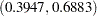. The lower categories indicate the favorable taste results; so Brand 1 scored significantly better when compared to Brand 2 or 3. Brand 2 scored less favorably when compared to Brand 3.

Figure 3 Multinomial Model Summary and Interval Statistics
The MCMC Procedure

Posterior Summaries
Parameter N Mean Standard
Deviation
Percentiles
25% 50% 75%
beta1 2500 0.3822 0.1337 0.2957 0.3811 0.4718
beta2 2500 -0.6502 0.1403 -0.7456 -0.6488 -0.5590
or12 2500 2.8366 0.4054 2.5518 2.8079 3.1088
or13 2500 1.4787 0.1982 1.3440 1.4638 1.6029
or23 2500 0.5271 0.0743 0.4745 0.5227 0.5718

Posterior Intervals
Parameter Alpha Equal-Tail Interval HPD Interval
beta1 0.050 0.1135 0.6443 0.1081 0.6344
beta2 0.050 -0.9297 -0.3736 -0.9357 -0.3828
or12 0.050 2.1196 3.6740 2.0851 3.6101
or13 0.050 1.1202 1.9047 1.1059 1.8741
or23 0.050 0.3947 0.6883 0.3849 0.6714

## References

Albert, J. H. and Chib, S. (1993), “Bayesian Analysis of Binary and Polychotomous Response Data,” Journal of the American Statistical Association, 88(422), 669–679.

McCullagh, P. and Nelder, J. A. (1989), Generalized Linear Models, Second Edition, London: Chapman & Hall.